The Stamping node inserts copies of a mask on a second mask. The node has two inputs: the base mask is linked to the top connector and the mask to use as the stamp to the second connector.

An optional mask connector allows a density mask to be used in the stamp. This option constrains the stamping to a part of the mask.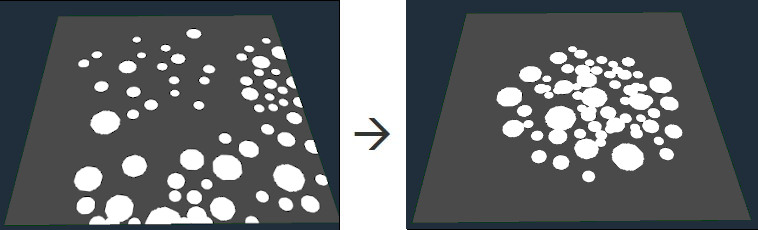To add a Stamping node, right-click in the Graph Editor and select Create Node  > Mask Composition  > Stamping.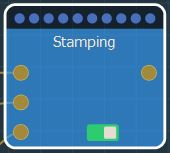Select the node to open its parameters: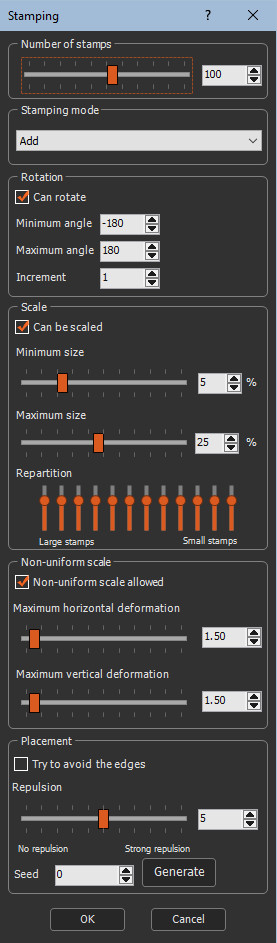### Setting the number of stamps

This parameter defines the number of stamps in the mask.

A larger value adds more stamps.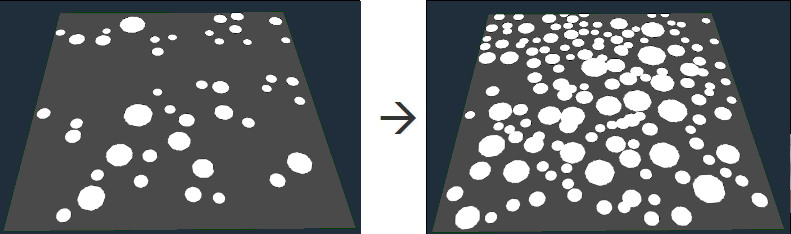### Setting the stamping mode

This parameter defines the how the stamp is placed in the mask.• Max: Adds the value of the stamp to the mask. When several stamps are at the same location, the maximum value of these stamps is used at each vertex.• Subtract: Subtracts the value of the stamp from the mask. The stamp is inverted and then added.• Min: Similar to Subtract, but when several stamps are at the same location, the minimum value of these stamps is used at each vertex.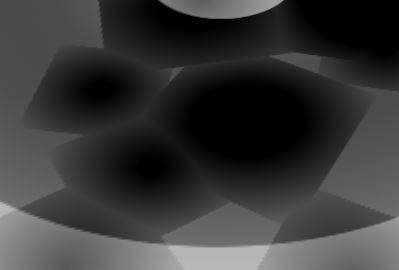### Setting the rotation

This parameter sets the angle of rotation of the stamp.

• Can rotate: When checked, the stamp can be rotated.
• Minimum angle: Sets the minimum rotation angle allowed down to -180°.
• Maximum angle: Sets the maximum rotation angle allowed up to 180°.
• Increment: Sets the minimum pace of rotation. Example with 45°.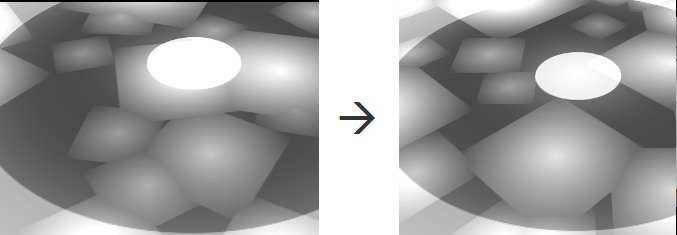### Setting the scaling

This parameter sets the percentage of scaling of the stamp and the repartition of the different sizes of stamps.

• Can be scaled: When checked, the stamp can be scaled.
• Minimum size: Sets the minimum allowed scale as a percentage of the stamp.
• Maximum size: Sets the maximum allowed scale as a percentage of the stamp.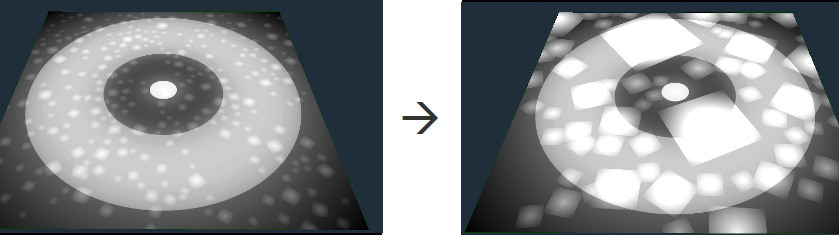• Repartition: Sets the distribution of large and small stamps using 10 vertical sliders corresponding to the different stamp sizes.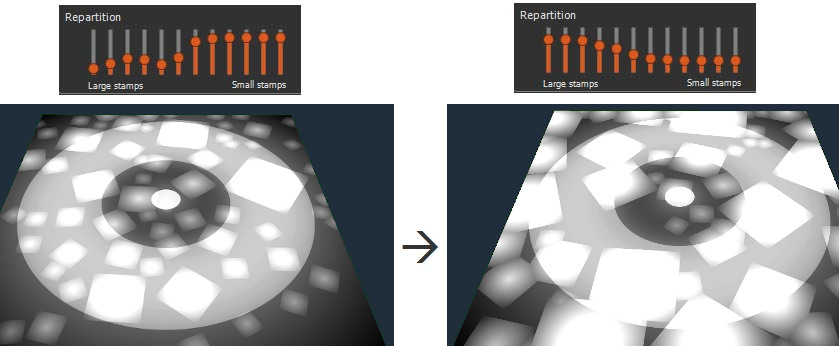To set the repartition to the default values, right-click and select Reset.

### Setting the non-uniform scaling

This parameter sets the non-uniform scaling along the horizontal and vertical axes.

• Non-uniform scale allowed: When checked, the stamp can be scaled non-uniformly.
• Maximum horizontal deformation: Sets the maximum horizontal deformation allowed, on a scale of 0 to 10.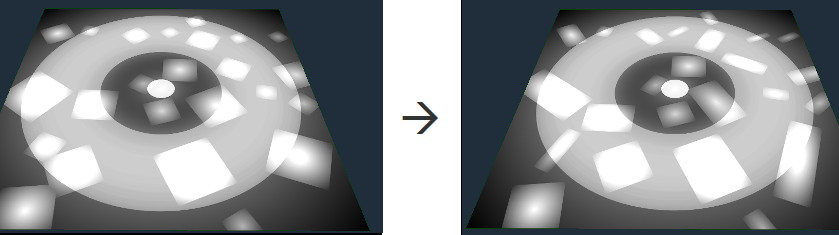• Maximum vertical deformation: Sets the maximum alteration of the values allowed, on a scale of 0 to 10.### Setting the placement

This parameter sets the distribution of the stamp.

Instant Terra tries to respect the density input mask as much as possible, but if the number of stamps is too high, there will be cases where the position of the stamps will not respect the density mask. In this case, the number of stamps or the repulsion must be reduced.

• Try to avoid the edges: When checked, the stamp is not constrained within the mask. The stamp can be placed on the mask border. this may not be respected when the number of stamps is too high.• Repulsion: Sets the distance between each stamp.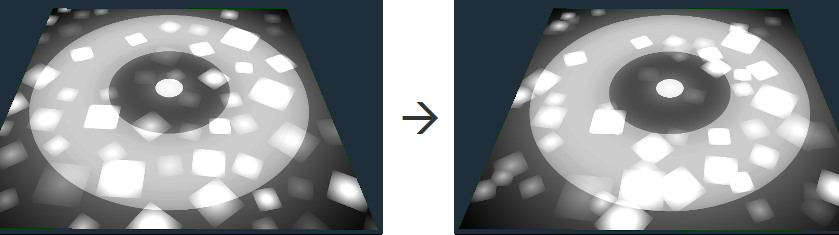• Seed: Value of the noise generation seed. Changing the value of the seed (even very slightly) completely changes the mask's appearance. Press Generate to vary the seed.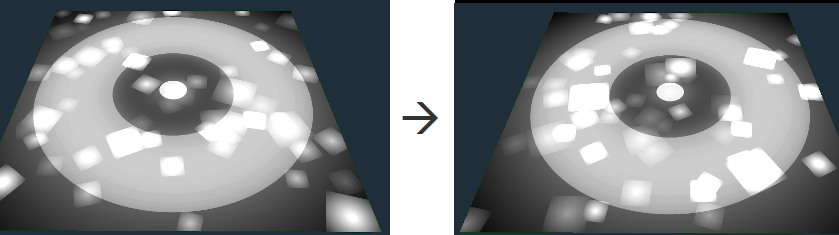### Parameters

Parameter Use
Number of stamps Defines the number of stamps in the mask.
Stamping mode Defines the how the stamp is placed in the mask.
Subtract Subtracts the stamp from the mask.
Min Similar to Subtract, but when several stamps are at the same location, the minimum value of these stamps is used at each vertex.
Max Adds the stamp to the mask to create a raised landscape. When several stamps are at the same location, the maximum value of these stamps is used at each vertex.
Rotation Sets the angle of rotation of the stamp.
Can rotate When checked, the stamp can be rotated.
Minimum angle Sets the minimum rotation angle down to -180°.
Maximum angle Sets the maximum rotation angle up to 180°.
Increment Sets the minimum angle of rotation.
Scale Sets the percentage of scaling of the stamp and the repartition of the different sizes of stamps.
Can be scaled When checked, the stamp can be scaled.
Minimum size Sets the minimum allowed scale as a percentage of the stamp.
Maximum size Sets the maximum allowed scale as a percentage of the stamp.
Repartition Sets the distribution of large and small stamps using 10 vertical sliders corresponding to the different stamp sizes.
Non-uniform scale Sets the non-uniform scaling along the horizontal and vertical axes.
Non-uniform scale allowed When checked, the stamp can be scaled non-uniformly.
Maximum horizontal deformation Sets the maximum horizontal deformation on a scale of 0 to 10.
Maximum vertical deformation Sets the maximum vertical deformation on a scale of 0 to 10.
Placement Sets the distribution of the stamp.
Try to avoid the edges When checked, the stamp is not constrained within the mask.
Repulsion Sets the distance between each stamp.
Seed Value of the noise generation seed.